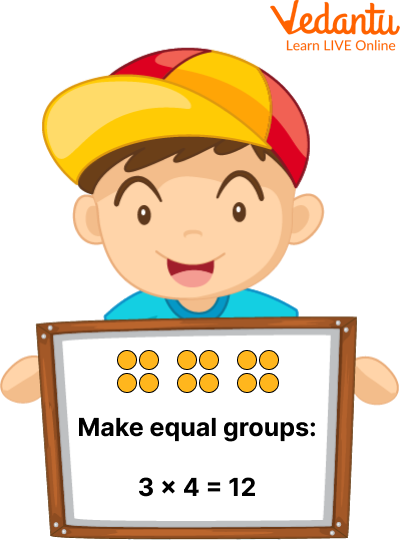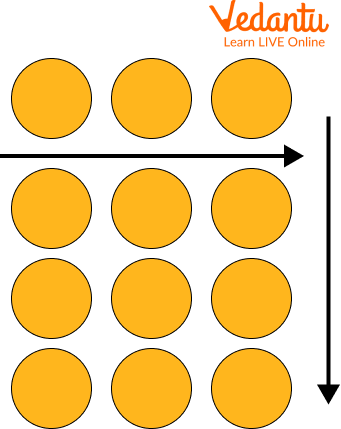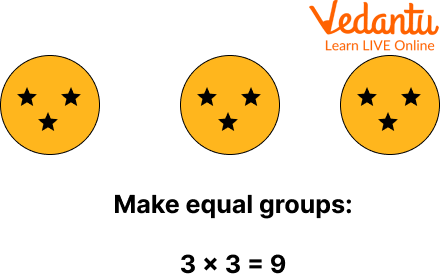Courses
Courses for Kids
Free study material
Free LIVE classes
MoreLIVE
Join Vedantu’s FREE Mastercalss

## Introduction to Multiplication Strategies

In our previous grades, we have read about the concept of multiplication. Let's revise with an example: you visit a bakery shop and buy 19 cakes for 3 rupees each. It's time to pay now, you will add 3 rupees to 19 times to get the final amount. Instead of addition here we can use the concept of multiplication. We are going to multiply $3 \times 19 =57$. So 57 is the final amount. In this article, we are going to learn about multiplication, how to teach multiplication and multiplication activities for class 3.

## What is Multiplication?

Multiplication is an operation that represents the basic idea of ​​adding the same number over and over again. Multiplied numbers are called factors, and the result obtained after multiplying two or more numbers is known as the product of those numbers. Multiplication is used to simplify the task of repeatedly adding the same number.

For example, what is 36 times 9? We know that 36 times 9 is written in the form of a multiplication sentence as 36 × 9 = 324. Here, 36 and 9 are the factors and 324 is the product. So, 36 times 9 is 324.

## Multiplication Formula

A multiplication expression is expressed as multiplicand × multiplier = product. Where:

• Multiplicand: first number (factor).

• Multiplier: Second number (factor).

• Product: The final result after multiplying the multiplicand and the multiplier.

• Multiplication symbol: "×" (connects the first number and second number).

## Multiplication Strategies for Grade 3

How to teach multiplication efficiently is important to help children understand multiplication. Teaching these four multiplication strategies for class 3 with the help of multiplication activity for class 3 is essential before children can remember facts.

### Make Equal Groups

One of the first multiplication strategies for 3rd grade is to start with equal grouping. This allows children to visually understand why two elements are multiplied to get a product.

It is helpful for children to start with manipulative devices such as yellow and red tokens. Start by writing the multiplication facts on your screen or blackboard.

In this example, we use 3x4.Equal Group

• Ask the children to form groups of three and place four game pieces in each group. Spend a few minutes creating groups and then discussing what they did. Ask children to share what they create.

• Explain that there are three groups and each has four counters. It's the same as saying 3×4. Ask the children to tell you the total number of game pieces they used.

• The answer is 12, as 3 × 4=12.

• This is a good time to point out that adding numbers gives you a total. Multiplication is the same.

### Make an Array

The next multiplication strategy I will teach you is to create an array: Creating a 3x4 array using a counter, similar to the group.

• So, create 3 rows with 4 counters in each row. Alternatively, you can create 4 rows of 3 counters each.

• When I teach an array, I don't care whether I display the rows or the columns first. Once we arrive at the commutativity of multiplication, we see that we can commute the factors anyway.

• It explains that creating three lines, including 4 in each line is the same as 3x4.

After using counters to create equal groups and arrays, let's move on to using numbers only. However, repeated addition can be combined with equal group and array formation.

Giving equal groups and formations is a useful strategy in the Army as it helps keep track of how many there are when counting totals.

When teaching repeated addition, tell students that it is easier to flip the factors. For example, if you find the product of 5 and 3, you can add 5+5+5 or 3+3+3+3+3. To show that counting by 5 is easier, he adds 5 three times.

### Skip Counting

Similar to repeated addition, skip counting can be used in conjunction with creating equal groups and arrays.

Students can label groups and skip how many people are in each group. This helps the students to keep track of their numbers.

The 4×3 example teaches students that they can skip 4 times 3 times or 3 times 4 times.

At the beginning of the school year, let students choose which strategy they like best.

Skipping numbers, however, is a strategy that children must use before they can memorize the facts of multiplication because this is the fastest strategy.

Sometimes you don't have time to draw a 9x8 array. So skipping the count is more efficient in this case.

## Solved Questions

Q1. Solve 3 x 4 using an array.

Ans: We use counters to create an array for 3×4. So we will create 3 rows with 4 counters in each row. Or we can have 4 rows with 3 counters in each.Using an Array

Thus, the result of the multiplication is 12.

Q2. Using repeated addition, solve 3 x 5.

Ans. Using repeated addition, we get,

3 + 3 + 3 + 3 + 3 or 15

Thus, the result of the multiplication is 15.

## Q3. Using an equal group, solve 3 x 3.

Ans. Using the concept of equal groups, we can transform the given product into 3 groups of 3 stars each, as shown below:Using Equal Group

Thus, the result of the multiplication is 9.

## Practice Questions

Q1. Multiply 30 x 2.

Ans. 60

Q2. Multiply 70 x 6.

Ans. 420

Q3. 6 x 9.

Ans. 54

Q4. 9 x 3.

Ans. 27

Summary

Last updated date: 02nd Oct 2023
Total views: 78.6k
Views today: 0.78k

## FAQs on Multiplication Strategies Grade 3

1. Who discovered matrix multiplication?

Matrix multiplication was first described by the French mathematician Jacques Philippe Marie Binet in 1812.

2. What is another name for skip counting?

This technique is also called counting by twos (threes, fours, etc.).

3. What sign can we use in place of the cross?

We can use * or . in the place of x in multiplication.